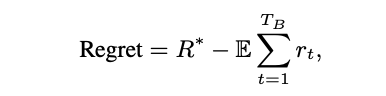# Multi-armed Bandits with ConstraintR∗ is the expected reward of the optimal policy (the policy that can obtain the maximum expected reward given the reward and cost distributions of each arm),
1. For reward- Beta(1,1)
2. For cost- Beta(1,1)
`import numpy as npimport randomfrom scipy.stats import betaimport matplotlib.pyplot as pltfrom numpy.random import choiceplt.rcParams['figure.figsize'] = [10, 7]import pandas as pddef plot_list(reward,penalties,iteration,plot_name):  x = np.linspace(0, 1, 10000)  y1 = beta.pdf(x, reward, penalties)  y2 = beta.pdf(x, reward, penalties)  y3 = beta.pdf(x, reward, penalties)  y4 = beta.pdf(x, reward, penalties)plt.title(f"PDF of Beta (Bell-shape) iteration({iteration} // {plot_name})", fontsize=20)  plt.xlabel("X", fontsize=16)  plt.ylabel("Probability Density", fontsize=16)  plt.plot(x, y1, linewidth=3, color='firebrick')  plt.annotate(f"Beta( {reward},{penalties}) { 10}", xy=(1.75, 19), size = 14, ha='center', va='center', color='firebrick')  plt.plot(x, y2, linewidth=3, color='burlywood')  plt.annotate(f"Beta( {reward},{penalties}) { 8}", xy=(1.75, 16.25), size = 14, ha='center', va='center', color='burlywood')  plt.plot(x, y3, linewidth=3, color='dodgerblue')  plt.annotate(f"Beta( {reward},{penalties}) { 5}", xy=(1.75, 15), size = 14, ha='center', va='center', color='dodgerblue')  plt.plot(x, y4, linewidth=3, color='green')  plt.annotate(f"Beta( {reward},{penalties}) { 3}", xy=(1.75, 17.5), size = 14, ha='center', va='center', color='green')  plt.ylim([0, 20])  plt.xlim([0, 2])  plt.show()def plot_count(num,iteration):  plt.bar(['A10','A08','A05','A03'],num)  plt.title(f"Arms selected the most  {iteration}")  plt.xlabel('Arms')  plt.ylabel('Number Of Times Each Arm Was Selected To Play')  plt.show()rl10 = [choice([0,0,1]) for i in range(4000)]rl8 =  [choice([0,0,1]) for i in range(4000)]rl5 =  [choice([0,1]) for i in range(4000)]rl3 =  [choice([0,0,1]) for i in range(4000)]data=pd.DataFrame({    'val10':rl10,    'val8':rl8,    'val5':rl5,    'val3':rl3,})rl10 = [choice([j/10 for j in range(10)]) for i in range(4000)]rl8 =  [choice([j/10 for j in range(10)]) for i in range(4000)]rl5 =  [choice([j/10 for j in range(10)]) for i in range(4000)]rl3 =  [choice([j/10 for j in range(10)]) for i in range(4000)]data_cost=pd.DataFrame({    'val10':rl10,    'val8':rl8,    'val5':rl5,    'val3':rl3,})def paper_approach_plot(observations,arms,data,data_cost):  #other initializations  Sr=*arms  Fr=*arms  Sc=*arms  Fc=*arms    arms_selected = []  numbers_of_selections_of_each_arm=*arms    for n in range(observations):    arm = 0    max_val = 0    for i in range(arms):      theta_r=random.betavariate(Sr[i]+1,Fr[i]+1)      theta_c=random.betavariate(Sc[i]+1,Fc[i]+1)      I=theta_r/theta_c      if I>max_val:            max_val=I            arm=i          r = data.values[n, arm]    c = data_2.values[n, arm]#     if r==0:#       c=0    Sr[arm]+=r    Fr[arm]+=(1-r)    Sc[arm]+=c    Fc[arm]+=(1-c)        arms_selected.append(arm)     numbers_of_selections_of_each_arm[arm]+=1if n%200==0 or n==observations-1:      plot_list(Sr,Fr,n,"reward")      plot_list(Sc,Fc,n,"cost")      plot_count(numbers_of_selections_of_each_arm,n)`